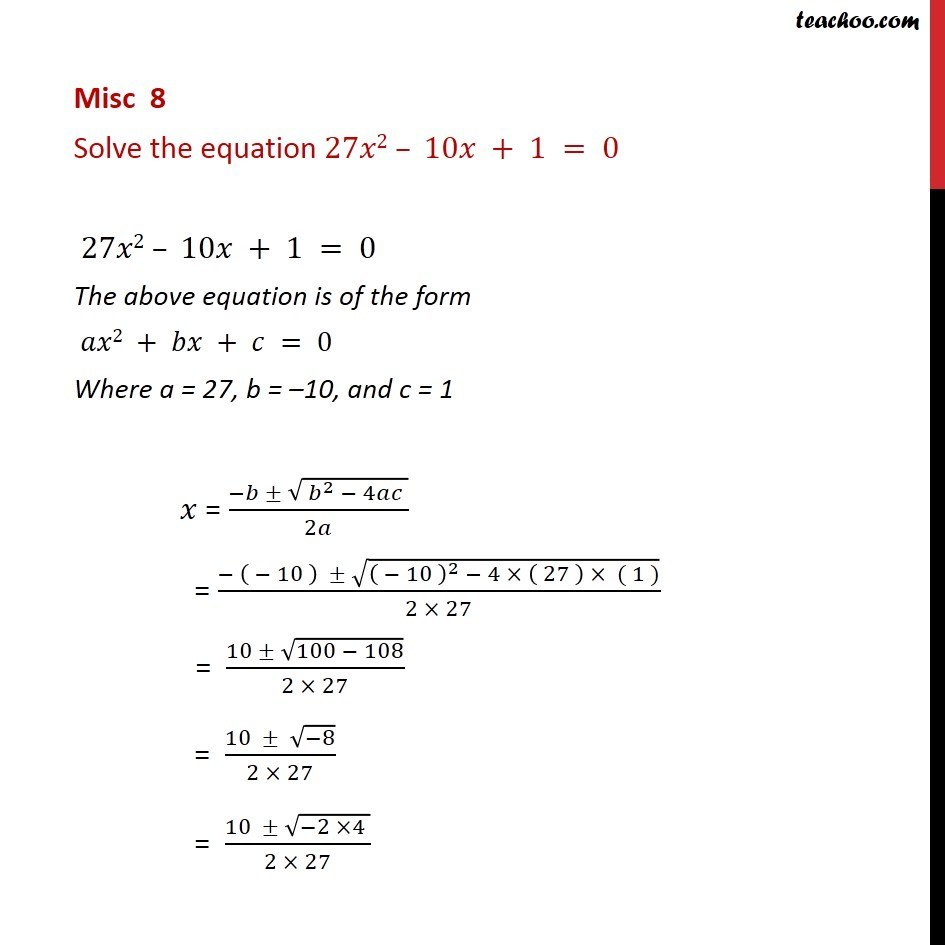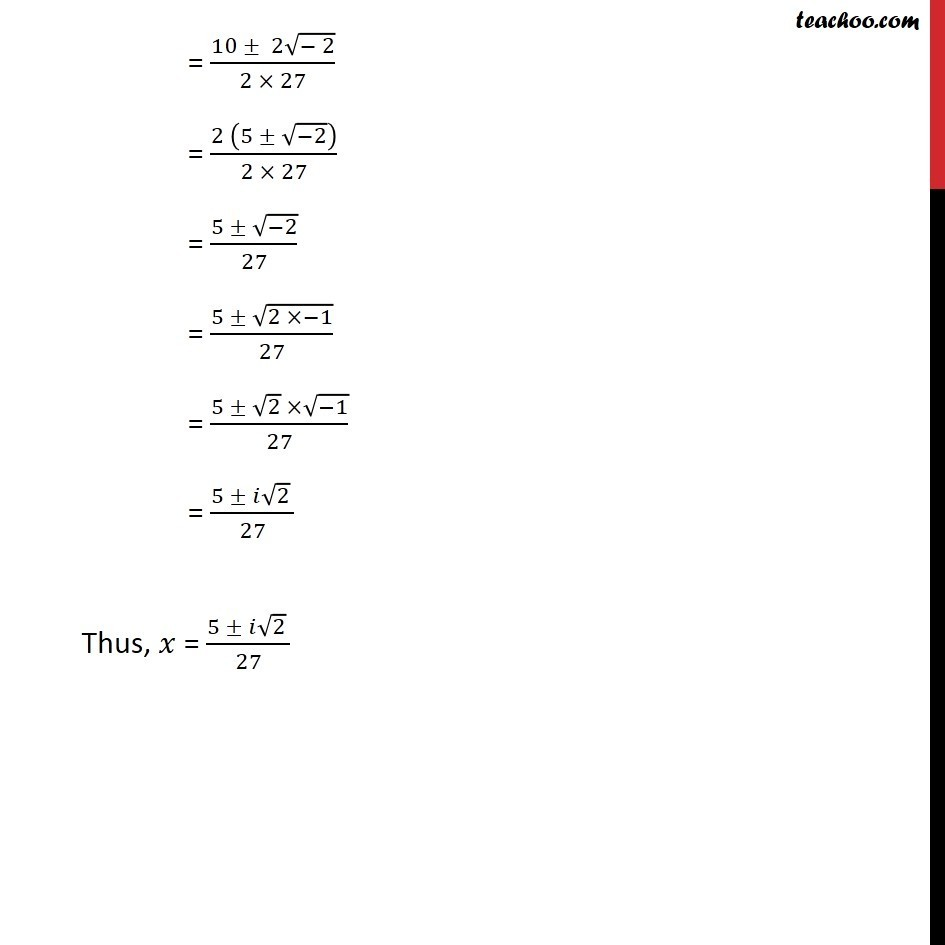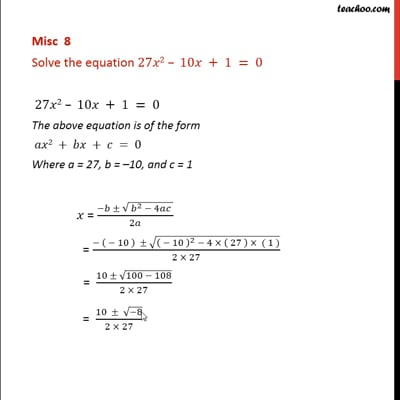Miscellaneous

Chapter 5 Class 11 Complex Numbers
Serial order wiseThis video is only available for Teachoo black users

Solve all your doubts with Teachoo Black (new monthly pack available now!)

### Transcript

Misc 8 Solve the equation 27 2 10 + 1 = 0 27 2 10 + 1 = 0 The above equation is of the form 2 + + = 0 Where a = 27, b = 10, and c = 1 = ( ( ^2 4 ))/2 = ( ( 10 ) (( 10 )^2 4 ( 27 ) ( 1 )))/(2 27) = (10 (100 108))/(2 27) = (10 ( 8))/(2 27) = (10 ( 2 4 ))/(2 27) = (10 2 ( 2))/(2 27) = (2 (5 ( 2)))/(2 27) = (5 ( 2))/27 = (5 (2 1))/27 = (5 2 ( 1))/27 = (5 2 )/27 Thus, = (5 2 )/27